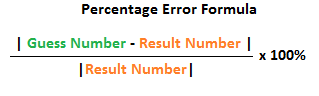# How to Calculate Percentage Error

Instructor: Allison Petrovic

Allison has experience teaching high school and college mathematics and has a master's degree in mathematics education.

We make guesses all the time - and we are not always correct! In this lesson, we will learn how to figure out the percentage error of an incorrect guess!

## How Tall are You?

Did you ever perform an experiment where, before you began, you had to make a guess of what the result would be? Imagine that you are in science class about to complete an experiment where you will be measuring your height! Let's say that your teacher told you to first guess how tall you are in inches and then figure out how far your guess is from the actual result. Sounds like a neat activity! In this height estimation experiment, your teacher is actually asking you to figure out the percentage error!

## What is Percentage Error?

The percentage error is a number, shown in percentage form, that explains how far away your guess is from the actual result. There is a neat formula that you can use to calculate the percentage error.Before we learn how to use the formula, you will need two important numbers: the guess number and the result number. So, for your height estimation experiment, you first need to make a guess as to how tall you are in inches! You guess 48 inches. Looking at the formula, you now just need the result number, which is your actual height. Your friend measures you and says you are 50 inches tall - this is our result. So, it appears as though you are a little taller than you guessed, right?!

## How to Calculate Percentage Error

Looking at the percentage error formula, it looks like you have all numbers that you will need! You have the guess number as 48 inches and the result number at 50 inches. Look at our Percentage Error Formula image and our Steps to Calculating Percentage Error image. Note that the little vertical lines surrounding the numerator and denominator are absolute value signs - they indicate that we are not concerned with whether or not any of the numbers are negative; instead, by using the absolute value, we will consider all numbers to be positive.

#### Steps to Calculate Percentage Error

Step 1: Plug in your numbers!

So you plug in 48 for guess number and 50 for result number.

Step 2: Subtract the result number from the guess number in the numerator.

So you have 48 inches minus 50 inches is equal to - 2 inches.

Step 3: Take the absolute value of the numerator and denominator.

To unlock this lesson you must be a Study.com Member.

### Register to view this lesson

Are you a student or a teacher?

#### See for yourself why 30 million people use Study.com

##### Become a Study.com member and start learning now.
Back
What teachers are saying about Study.com

### Earning College Credit

Did you know… We have over 200 college courses that prepare you to earn credit by exam that is accepted by over 1,500 colleges and universities. You can test out of the first two years of college and save thousands off your degree. Anyone can earn credit-by-exam regardless of age or education level.